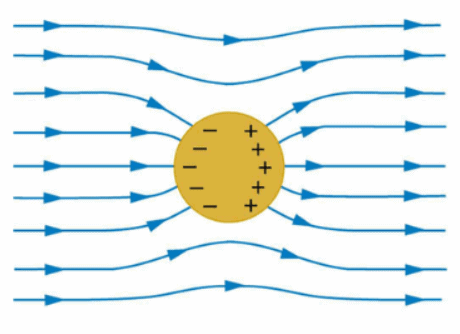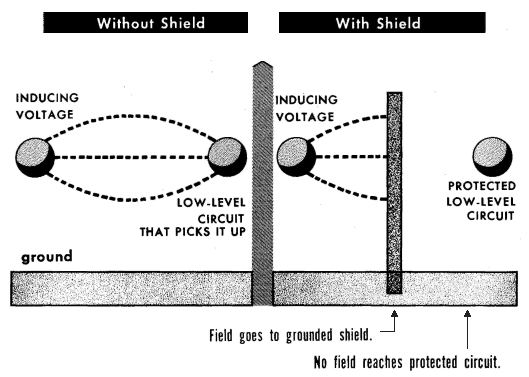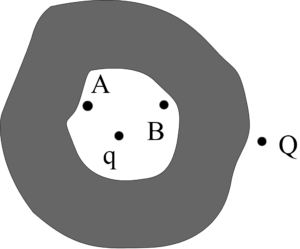Courses

# Electrostatics of Conductors Class 12 Notes | EduRev

## Class 12 : Electrostatics of Conductors Class 12 Notes | EduRev

The document Electrostatics of Conductors Class 12 Notes | EduRev is a part of the Class 12 Course Physics For JEE.
All you need of Class 12 at this link: Class 12

Electrostatic Properties of Conductors

• Conductors contain mobile charge carriers. In metallic conductors, these charge carriers are electrons. In a metal, the outer (valence) electrons part away from their atoms and are free to move. These electrons are free within the metal but not free to leave the metal.• In an external electric field, they drift against the direction of the field. The positive ions made up of the nuclei and the bound electrons remain held in their fixed positions. In electrolytic conductors, the charge carriers are both positive and negative ions. Some of the important points about the electrostatic properties of a conductor are as follows:

1. The electrostatic field is zero inside a conductor

• In the static condition, whether a conductor is neutral or charged, the electric field inside the conductor is zero everywhere. This is one of the defining properties of a conductor.
• We know that a conductor contains free electrons which, in the presence of an electric field, experience a drift or a force. Inside the conductor, the electrons distribute themselves in such a way that the final electric field at all points inside the conductor is zero.

2. Electrostatic field lines are normal to the surface at every point in a charged conductor

• We can say, if the electric field lines were not normal at the surface, a component of the electric field would have been present along the surface of a conductor in the static condition.
• Thus, free charges moving on the surface would also have experienced some force leading to their motion. But, this does not happen. Since there are no tangential components, the forces have to be normal to the surface.

3. In static conditions, the interior of the conductor contains no excess charge

• We know that any neutral conductor contains an equal amount of positive and negative charges, at every point. This holds true even in an infinitesimally small element of volume or surface area.
• From the Gauss’s law, we can say that in the case of a charged conductor, the excess charges are present only on the surface.
• Let us consider an arbitrary volume element of the conductor, which we denote as ‘v’ and for the closed surface bounding the volume element, the electrostatic field is zero. Thus, the total electric flux through S is zero. So, from the Gauss law, it follows that the net charge enclosed by the surface element is zero.
• As we go on decreasing the size of the volume and the surface element, at a point we can say that when the element is vanishingly small, it denotes any point in the conductor. So the net charge at any point inside the conductor is always zero and the excess charges reside at the surface.

4. Constant electrostatic potential throughout the volume of the conductor

• The electrostatic potential at any point throughout the volume of the conductor is always constant and the value of the electrostatic potential at the surface is equal to that at any point inside the volume.

5. Electrostatic Shielding

• The third kind of shielding is a protection against electric induction, commonly called electrostatic induction. (Electrostatic induction is really a misnomer; this induction is better called electric induction, because it depends upon the continuous fluctuation of the charges induced.)Electrostatic Shielding

• Electric shielding consists of interposing a grounded shield between the interfering voltage and the low-level circuit that might pick up the electric field. The voltage induced is immediately carried off to ground, and the electric field is prevented from reaching the circuit that is shielded.
• Unlike either form of protection against magnetic fields, shielding against electric fields can be almost 100% effective. All that is necessary is to insure that no path is left through which the electric field can pass. For this reason, much more attention is given to the prevention of induction by magnetic fields.

Solved Example
Assertion: A point charge is placed inside a cavity of the conductor as shown. Another point charge Q is placed outside the conductor as shown. Now as the point charge Q is pushed away from the conductor, the potential difference (VA – VB) between two points A and B within the cavity of sphere remains constant.

Reason: The electric field due to charge on the outer surface of the conductor and outside the conductor is zero at all points inside the conductorA. Both Assertion and Reason are correct and the Reason is the correct explanation for Assertion

B. Both Assertion and Reason are correct and the Reason is not the correct explanation for Assertion

C. Assertion is correct but Reason is incorrect

D. Assertion is incorrect but Reason is correct
Solution:
Option A. As we know that that the electric field inside the conductor is zero, so the field inside the conductor is constant. Therefore the potential between point A and B will remain constant.

Offer running on EduRev: Apply code STAYHOME200 to get INR 200 off on our premium plan EduRev Infinity!

## Physics For JEE

187 videos|516 docs|263 tests

,

,

,

,

,

,

,

,

,

,

,

,

,

,

,

,

,

,

,

,

,

;